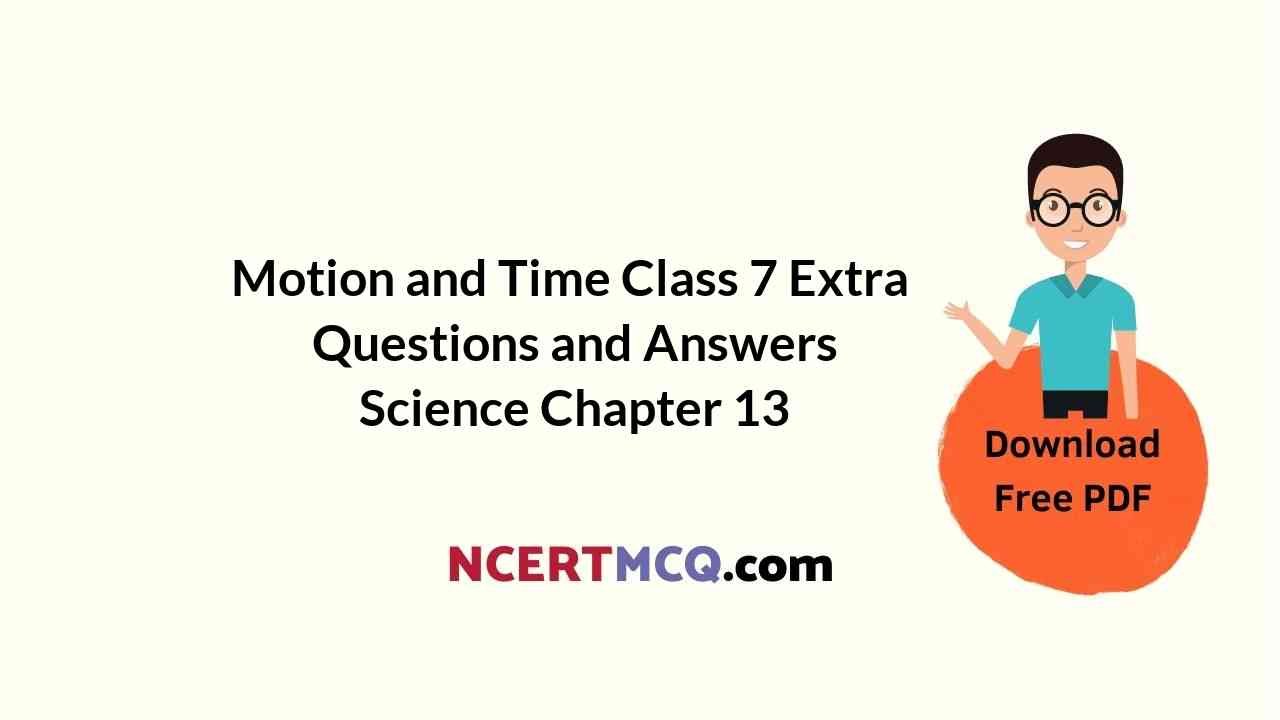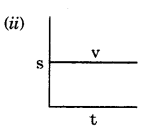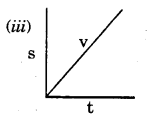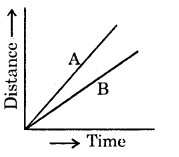Here we are providing Online Education Motion and Time Class 7 Extra Questions and Answers Science Chapter 13 was designed by subject expert teachers. https://ncertmcq.com/extra-questions-for-class-7-science/

## Online Education for Class 7 Science Chapter 13 Extra Questions and Answers Motion and Time

Motion And Time Class 7 Extra Questions With Answers Question 1.
Write formula for speed.Class 7 Science Chapter 13 Extra Questions Question 2.
Define a day.
The time between one sunrise and the next is called a day.

Class 7 Motion And Time Extra Questions Question 3.
What is a year?
A year is fixed as the time taken by the earth to complete one revolution around the sun.

Motion And Time Class 7 Questions With Answers Question 4.
What is a month?
A month is measured from one new moon to the next.Chapter 13 Science Class 7 Extra Questions Question 5.
What is bob?
The metallic ball hanging with the thread is called the bob of the pendulum.

Motion And Time Class 7 Questions And Answers Question 6.
What is oscillatory motion?
The to and fro motion of an object is called periodic or an oscillatory motion of that object.

Class 7 Science Ch 13 Extra Questions Question 7.
What is speedometer?
It is a device that is used to measure speed directly in km/h.

Time And Motion Class 7 Questions And Answers Question 8.
What is odometer?
Meter that measures the distance moved by the vehicle is known as an odometer.

Ncert Class 7 Science Chapter 13 Extra Questions Question 9.
“Speed of a car is 50 km/h.” What does it mean?
When we say that a car is moving with a speed of 50 kilometres per hour, it implies that it will cover a distance of 50 kilometres in one hour.

Ncert Solutions For Class 7 Science Chapter 13 Extra Questions Question 10.
What are microsecond and nano-second?
One microsecond is one-millionth of a second. A nanosecond is one-billionth of a second.

Class 7 Science Motion And Time Extra Questions Question 11.
Name different types of graphs.
Bar graph, line graph, pie graph.Motion And Time Extra Questions Question 12.
How is speed of car in non-uniform motion measured?
The speed is calculated as the total distance covered divided by the total time taken. Thus,This is called average speed.

Motion And Time Class 7 Extra Questions Question 13.
Suggest some points to be kept in mind while choosing the most suitable scale for drawing a graph.
Some of the points to be kept in mind while choosing the most suitable scale for drawing a graph are :

• the difference between the highest and the lowest values of each quantity.
• the intermediate values of each quantity, so that with the scale chosen it is convenient to mark the values on the graph, and
• to utilise the maximum part of the paper on which the graph is to be drawn.

Time And Motion Class 7 Extra Questions Question 14.
A ball moves 30 m in 6 s. Find its speed.
Here, d = 30 m
t = 6 s
s = ?
We know, s = $$\frac{d}{t}$$
= $$\frac{30 \mathrm{~m}}{6 \mathrm{~s}}$$ = 5 ms<sup>-1</sup>.

Class 7 Science Chapter 13 Extra Question Answer Question 15.
A body is moving with a speed 10 m/s. After 1 minute, what is the distance travelled?
Here, s = 10 m/s
t = 1 min = 60 s
d =?
We know,
s = $$\frac{d}{t}$$
⇒ d =s.t.
= 10 m/s × 60 s
=600m.

Questions On Motion And Time Class 7 Question 16.
Find the distance covered by a car moving at 50 kmlh in 2 hours.
Given, s = 50 km/h
t=2h
d=?
We know that,
s =$$\frac{d}{t}$$
or, d=st
=5okm/h×2h
=100km.Extra Questions On Motion And Time For Class 7 Question 17.
The bowling speed of Shoaib Akhtar is 144 km/h. Find in what time the ball reaches the batsman. Consider that the batsman is standing at a distance 20 m from the bowling crease.
Given, s = 144 km/h
= $$144 \times \frac{5}{18}$$m/s
[∵1 km/hr = $$\frac{5}{18}$$m/s]
= 40 in/s
d=20m

We know that,
s = $$\frac{d}{t}$$
or, t=$$\frac{d}{s}$$
= [layex]\frac{20 \mathrm{~m}}{40 \mathrm{~m} / \mathrm{s}}[/latex] = $$\frac{1}{2}$$s = 0.5 s

Class 7 Science Motion And Time Question Answers Question 18.
Write briefly about the development of pendulum clock.
It is said that once Galileo (AD 1564-1642) was sitting in a church. He noticed that a lamp suspended from the ceiling with a chain was moving slowly from one side to the other. He was surprised to find that his pulse beat the same number of times during the interval in which the lamp completed one oscillation.

Galileo experimented with various pendulums to verify his observation. He found that a pendulum of a given length takes always the same time to omp1ete one oscillation. This observation led to the development of pendulum clocks. Winding clocks and wristwatches were refinements of the pendulum clocks.

Extra Questions Of Motion And Time Class 7 Question 19.
A car moves with a speed of 40 km/h for 15 minutes and then with a speed of 60 km/h for the next 15 minutes. The total distance covered by the car is:
(i) 100km
(ii) 25km
(iii) 15 km
(iv) 10 km
Distance travelled in first 15 min
= speed × time
= 40 km/h × 15 min
= $$\frac{40 \mathrm{~km}}{\mathrm{~h}} \times \frac{15}{60} \mathrm{~h}$$ = 10 km

Distance travelled in last 15 min
= speed × time
= 60 km/h × 15 min
=60km/h × $$\frac{15}{60}$$ h=15 km
∴ Total distance = (10 + 15) km = 25 km.

Motion And Time Class 7 Questions Question 20.
Salma takes 15 minutes from her house to reach her school\on a bicycle. If the bicycle has a speed of 2 m/s, calculate
the distance between her house and the school.
Speed = 2 m/s
Time = 15 min = 15 × 60’s
= 900 s.
∴ Distance = speed × time
= 2 m/s × 900 s = 1800 m
= $$\frac{1800}{1000}$$km = 1.8 km.Question 21.
The odometer of a car reads 57,321.0 km when the clock shows the time 08:30 AM. What is the distance moved by the car, if at 08:50 AM, the odometer reading has changed to 57,336.0 km? Calculate the speed of the car in km/mm during this time. Express the speed in km/h also.
Odometer reading at 8:30 am = 57321.0 km
Odometer reading at 8:50 am = 57336.0 km
∴ Distance travelled = (57336.0 – 57321.0) km
= 15.0 km.

T time taken = 8:50 am – 8:30 am = 20 min
∴Speed = $$\frac{\text { distance }}{\text { time }}=\frac{15 \mathrm{~km}}{20 \mathrm{~min}}$$ = 0.75 km/mm
∴ Speedinkm/h=$$\frac{0.75 \mathrm{~km}}{\frac{1}{60} \mathrm{~h}}$$
(∴ 1 min=$$\frac{1}{60}$$h)
= 0.75 x 60 km/b
= 45 km/h.

Question 22.
Can you also find the speed of the bus from its distance-time graph?
Distance travelled = 80 km.
Time required = 10:00 am – 8:00 am =2h= $$\frac{80 \mathrm{~km}}{2 \mathrm{~h}}$$ = 40 km/h.

Question 23.
The distance between two stations is 240 km. A train takes 4 hours to cover this distance. Calculate the speed of the train.
Distance = 240 km
Time = 4 h
∴ Speed =$$\frac{\text { distance }}{\text { time }}=\frac{240 \mathrm{~km}}{4 \mathrm{~h}}$$
= 60 km/h.

Multiple Choice Questions

1. What is the relationship between distance, time and speed?
(i) Speed =$$\frac{\text { Distance }}{\text { Time }}$$
(ii) Time = $$\frac{\text { Speed }}{\text { Distance }}$$
(iii) Distance = $$\frac{\text { Speed }}{\text { Time }}$$
(iv) Distance = $$\frac{\text { (Speed) }^{2}}{\text { Time }}$$
(i) Speed =$$\frac{\text { Distance }}{\text { Time }}$$.2. The graph for uniform speed in distance-time graph is : (s = distance, t =time, u = speed)3. Which of the following factors affects the time period of the pendulum?
(i) Length of string
(ii) Mass of bob
(iii) Initial displacement of the bob
(iv) Gravity value
(i) Length of string.

4. Which of the following is not a unit of time?
(i) Second (s)
(ii) Minutes (mm)
(iii) Hours (h)
(iv) Hertz (Hz).
(iv) Hertz (Hz).

5. Which of the following is not a unit
of speed?
(i) m/s
(ii) m/mm
(iii) m/Hz
(iv)km/h
(iii) m/Hz.6. For converting km/h to m/s, we have to multiply magnitude of km/h with :
(i) $$\frac{1000}{60}$$
(ii) $$\frac{1000}{60^{2}}$$
(iii) $$\frac{100}{60}$$
(iv) $$\frac{100}{60^{3}}$$.
(ii) $$\frac{1000}{60^{2}}$$.

7. Speed measuring device of a vehicle is known as :
(i) Odometer
(ii) Speedometer
(iii) Aurometer
(iv) None of these.
(ii) Speedometer.

8. Distance travelled by a vehicle is measured by :
(i) Odometer
(ii) Speedometer
(iii) Aurometer
(iv) Barometer.
(i) Odometer.

9. The to and fro motion of pendulum is known as :
(i) uniform motion
(ii) non-uniform motion
(iii) oscillation
(iv) frequency motion.
(iii) oscillation.

10. Distance-time graph gives :
(i) speed
(ii) displacement
(iii) frequency
(iv) acceleration.
(i) speed.11. Figure below shows the distance-time graph for the motion of two vehicles A and BWhat can you conclude from this graph?
(i) A is moving faster than B
(ii) B is moving faster than A
(iii) Both are moving with same speed
(iv) Data is insufficient to conclude anything
(i) A is moving faster than B.

Keywords:

→ Bar graph: A graph in which data are represented by columns is called a bar graph.

→ Graphs: Pictorial representations of data are called graphs.

→ Non-uniform motion: If the speed of an object moving along a straight line keeps changing, its motion is said to be non-uniform.

→ Oscillation: The back and forth movement of an object is termed as oscillation of that object.

→ Simple Pendulum: A simple pendulum consists of a small metallic ball or a piece of stone suspended from a rigid stand by a thread.→ Speed: The distance covered by an object in unit time is called speed of that object.

→ Time period: The time taken by the pendulum to complete one osçjllation is called its time period.

→ Uniform motion: An object moving along a straight line with a constant speed is said to be in uniform motion.

→ Unit of time: The SI unit of time is second.